# NCERT Solutions For Class 6 Maths Ratios and Proportions Exercise 12.2

NCERT Solutions For Class 6 Maths Ratios and Proportions Exercise 12.2

### NCERT Solutions For Class 6 Maths Ratios and Proportions Exercise 12.2

NCERT Solutions For Class 6 Maths Chapter 12 Ratios and Proportions Ex 12.2

Exercise 12.2

Ex 12.2 Class 6 Maths Question 1.
Determine if the following are in proportion,
(a) 15, 45, 40, 120
(b) 33, 121, 9, 96
(c) 24, 28, 36, 48
(d) 32, 48, 70, 210
(e) 4, 6, 8, 12
(f) 33, 44, 75, 100
Solution: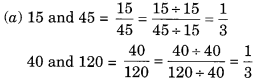∴ 15 : 45 :: 40 : 120
∴ 15, 45, 40 and 120 are in proportion.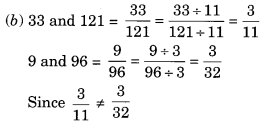∴ 33, 121, 9 and 96 are in proportion.∴ 24, 28, 36 and 48 are not in proportion.Since 2/3 ≠ 1/3
∴ 32, 48, 70 and 210 are not in proportion. 4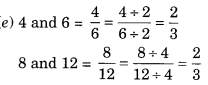∴ 4 : 6 :: 8 : 12
∴ 4, 6, 8 and 12 are in proportion.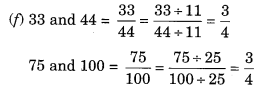∴ 33 : 44 : : 75 : 100
∴ 33, 44, 75 and 100 are in proportion.

Ex 12.2 Class 6 Maths Question 2.
Write True (T) or False (F) against each of the following statements:
(a) 16 : 24 :: 20 : 30
(b) 21 : 6 :: 35 : 10
(c) 12 : 18 :: 28 : 12
(d) 8 : 9 :: 24 : 27
(e) 5.2 : 3.9 :: 3 : 4
(f) 0.9 : 0.36 :: 10 : 4
Solution:
(a) 16 : 24 :: 20 : 30
Product of the extreme terms = 16 x 30 = 480
Product of the middle terms = 24 x 20 = 480
∴ The given statement (a) → (T)

(b) 21 : 6 :: 35 : 10
Product of the extreme terms = 21 x 10 = 210
Product of the middle terms = 6 x 35 = 210
∴ The given statement (b) → (T)

(c) 12 : 18 :: 28 : 12
Product of the extreme terms = 12 x 12 = 144
Product of the middle terms = 18 x 28 = 504
Since 144 ≠ 504
∴ The given statement (c) → (F)

(d) 8 : 9 :: 24 : 27
Product of the extreme terms = 8 x 27 = 216
The product of the middle terms = 9 x 24 = 216
The given statement (d) → (T)

(e) 5.2 : 3.9 :: 3 : 4
Product of the extreme terms = 5.2 x 4 = 20.8
Product of the middle terms = 3.9 x 3 = 11.7
Since 20.8 ≠ 11.7
The given statement (e) → (F)

(f) 0.9 : 0.36 :: 10 : 4
Product of the extreme terms = 0.9 x 4 = 3.6
Product of the middle terms = 0.36 x 10 = 3.6
∴ The given statement (f) → (T)

Ex 12.2 Class 6 Maths Question 3.
Are the following statements true?
(a) 40 persons : 200 persons = ₹15 : ₹75
(b) 7.5 litres : 15 litres = 5 kg : 10 kg
(c) 99 kg : 45 kg = ₹ 44 : ₹ 20
(d) 32 m : 64 m = 6 sec : 12 sec
(e) 45 km : 60 km = 12 hours : 15 hours
Solution:
(a) 40 persons : 200 persons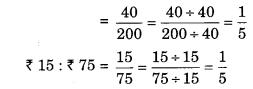∴ Statement (a) is true.

(b) 7.5 litres : 15 litres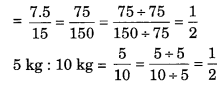∴ Statement (b) is true.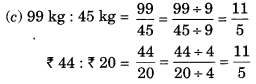∴ Statement (c) is true.∴ Statement (d) is true.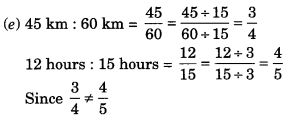∴ Statement (e) is not true.

Ex 12.2 Class 6 Maths Question 4.
Determine if the following ratios form a proportion. Also, write the middle terms and extreme terms where the ratios form a proportion.
(a) 25 cm : 1 m and ₹ 40 : ₹ 160
(b) 39 litres : 65 litres and 6 bottles : 10 bottles
(c) 2 kg : 80 kg and 25 g : 625 g
(d) 200 mL : 2.5 litres and ₹ 4 : ₹ 50
Solution:
(a) 25 cm : 1 m = 25 cm : 100 cm [∵ 1 m = 100 cm]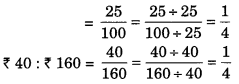∴ The given ratios are in proportion.
Extreme terms are 25 cm and ₹ 160.
Middle terms are 1 m and ₹40.∴ The given ratios are in proportion.
Extreme terms are 39 litres and 10 bottles.
Middle terms are 65 litres and 6 bottles.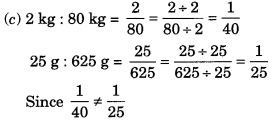∴ The given ratios are not in proportion.

(d) 200 mL : 2.5 litres = 2.5 litres = 2.5 x 1000 mL = 2500 mL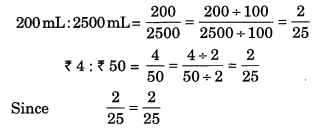∴ The given ratios are in proportion.
Extreme terms are 200 mL and ₹ 50
Middle terms are 2.5 litres and ₹ 4.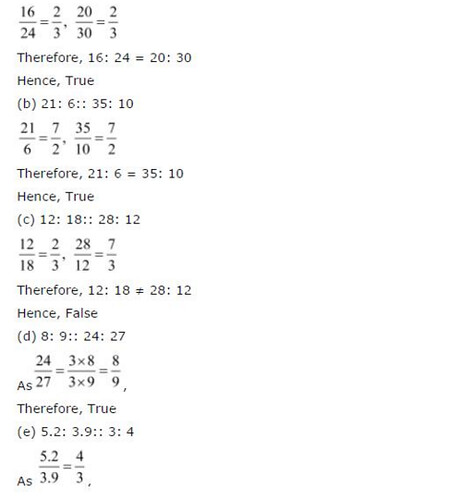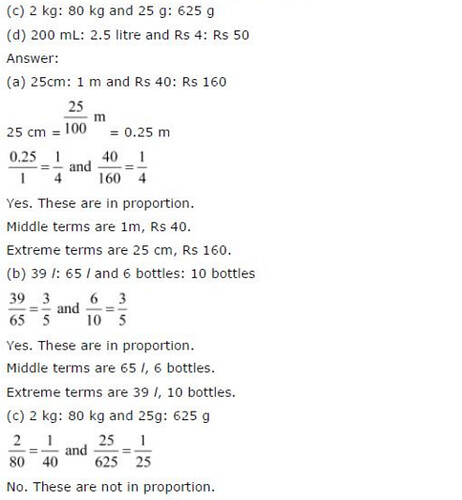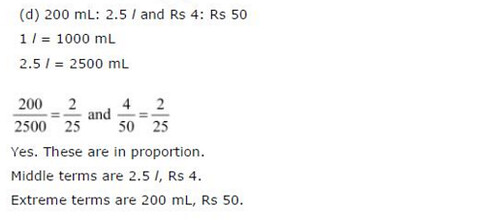## SabDekho

The Complete Educational Website